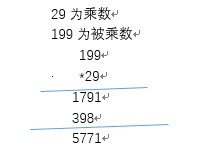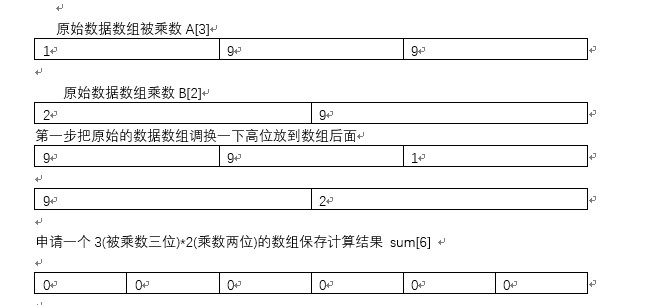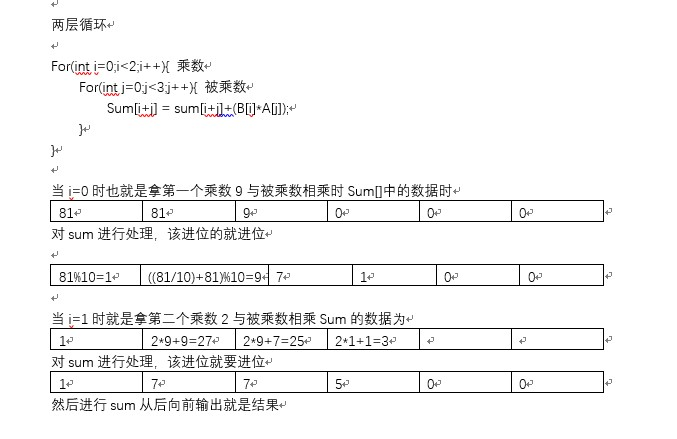• 前几天在网上看到这样一个题目 “数相乘，小数点后的位数没有限制，写一个高效算法“，在网上搜集到一些想法，感觉”先做大数乘法，然后再给结果加小数点“这种方法更好一些，用c语言实现了，分享给大家。...
前几天在网上看到这样一个题目 “两个数相乘，小数点后的位数没有限制，写一个高效算法“，在网上搜集到一些想法，感觉”先做大数乘法，然后再给结果加小数点“这种方法更好一些，用c语言实现了，分享给大家。如果大家有更好的方法，欢迎一起交流。
#include<stdio.h>
#include<string.h>

void longmulti(const char *a, const char *b, char *c);
void decimalmulti(char *a, char *b, char *c);

int main(void)
{
char c;
decimalmulti("3.1415926", "3.14", c);
printf("3.1415926 * 3.14 = %s\n\n", c);
return 0;
}

void longmulti(const char *a, const char *b, char *c)
{
int i, j, k, n, carry;
int len_a, len_b;

i = j = k = 0;
//如果a，b中有一个是0，则整个运算的结果为0
if(!strcmp(a, "0") || !strcmp(b, "0"))
{
c = '0';
c = '\0';
return;
}
//判断运算结果的正负号
if(a == '-')
{
i = 1;
k = !k;
}
if(b == '-')
{
j = 1;
k = !k;
}
//如果出现了符号，先将符号过滤掉
if(i || j)
{
if(k)
c = '-';
longmulti(a+i, b+j, c+k);
return;
}
len_a = strlen(a);
len_b = strlen(b);

memset(c, '0', len_a + len_b);
c[len_a + len_b] = '\0';
//各位数的运算
#define I(a) (a - '0')
for(i = len_a - 1; i >= 0; i--)
{
for(j = len_b - 1, k = i+j+1, carry = 0; j >= 0; j--, k--)
{
n = I(a[i]) * I(b[j]) + I(c[k]) + carry;
c[k] = (n % 10) + '0';
carry = n / 10;
}
c[k] += carry;
}
#undef I
if(c == '0')
memmove(c, c+1, len_a + len_b);
}

void decimalmulti(char *a, char *b, char *c)
{
/*
len_a记录字符串a的长度
len_b记录字符串b的长度
len_c记录字符串c的长度
dot_a记录小数点在字符串a中的位置，从1开始计数，即数组下标加1。
dot_b记录小数点在字符串b中的位置。
index记录相乘后的结果中应该有几位小数
*/
int len_a, len_b, len_c, i, j, k, dot_a, dot_b, index;

len_a = strlen(a);
len_b = strlen(b);
dot_a = len_a;//小数点的位置为字符串的长度，表示不存在小数点
dot_b = len_b;

//将字符串a中的小数点去掉，并记录小数点的位置
for(i = 0, k = 0; i < len_a; i++)
{
if(a[i] == '.')
dot_a = i + 1;//在数组中的索引+1，为了方便后面计算
else
a[k++] = a[i];
}
a[k] = '\0';
//将字符串b中的小数点去掉，并记录小数点的位置
for(i = 0, k = 0; i < len_b; i++)
{
if(b[i] == '.')
dot_b = i + 1;
else
b[k++] = b[i];
}
b[k] = '\0';

//计算相乘后的结果总共有多少位小数
index = (len_a - dot_a) + (len_b - dot_b);
//大整数乘法
longmulti(a, b, c);
len_c = strlen(c);
//为计算后的结果添加小数点
if(index > 0)
{
for(i = len_c; i >= len_c - index; i--)
c[i + 1] = c[i];
c[len_c - index] = '.';
}

//过滤左边的0
len_c = strlen(c);
for(i = 0, j = 0; i < len_c; i++)
{
if(c[i+1] == '.' || c[i] != '0')
break;
j++;
}
if(j)
memmove(c, c+j, len_c - j + 1);
//过滤右边的0
len_c = strlen(c);
for(i = len_c - 1; i >= 0; i--)
if(c[i] == '0')
c[i] = '\0';
else if(c[i] == '.')
{
c[i] = '\0';
break;
}
else
break;
}


展开全文c 语言
• 带有符号的多位数相乘 以199×29为例 基本时执行步骤如下图 完整代码 #include &amp;lt;stdio.h&amp;gt; #include &amp;lt;string.h&amp;gt; #include&amp;lt;stdlib.h&amp;gt; #define ...
带有符号的多位数相乘
以199×29为例基本时执行步骤如下图完整代码
#include <stdio.h>
#include <string.h>
#include<stdlib.h>
#define NUM 64 //定义最大的位数

//计算操作 操作函数
void count(const char *data1,const char* data2,int*sum){
int lendata1 = strlen(data1);
int lendata2 = strlen(data2);
int i,j;
int flag = 0;
int num;
//进行相乘
for(i=0;data2[i];i++){ //乘数
for(j=0;data1[j];j++){//被乘数
sum[i+j] = sum[i+j]+((data2[i]-'0')*(data1[j]-'0'));
}
//对结果进行处理，该进位的进位
flag = 0;
for(j=0;j<2*NUM;j++){
num = sum[j]+flag;
flag = num/10;
sum[j] = num%10;
}

}

}
//进行数组反转
void  translot(char * str)
{
int len = strlen(str);
int i;
for(i=0;i<len/2;i++)
{
char tem = str[i];
str[i] = str[len-1-i];
str[len-1-i] = tem;
}

}

void main(void)
{
char data1;
char data2[NUM+1];
int sum[2*NUM+1]={0};
int flag1=1;
int flag2 = 1;
printf("请输入数据1:");
scanf("%s",data1);
while(strlen(data1)>NUM)
{
printf("请输入低于%d位的数据1:",NUM);
scanf("%s",data1);
}
printf("请输入数据2:");
scanf("%s",data2);

while(strlen(data2)>NUM)
{
printf("请输入低于于%d位的数据2:",NUM);
scanf("%s",data2);
}
//判断输入的数据是否带有符号
if(data1=='-'){
flag1=-1;
char *tem =  strtok(data1,"-");
strcpy(data1,tem);
}else if(data1=='+')
{
char *tem = strtok(data1,"+");
strcpy(data1,tem);
}

if(data2=='-'){
flag2=-1;
char*tem = strtok(data2,"-");
strcpy(data2,tem);
}else if(data2=='+'){
char *tem  = strtok(data2,"+");
strcpy(data2,tem);
}
//把数组反转
translot(data1);
translot(data2);
//进行计算
count(data1,data2,sum);
//过滤结果数组后面的0
int i=2*NUM;
for(;i>=0;i--)
{
if(sum[i]!=0)
break;
}
//打印符合
if(flag1*flag2<0){
printf("-");
}
for(i;i>=0;i--)
{
printf("%d",sum[i]);
}
printf("\n");
}



展开全文• 两数相乘溢出的问题 将两个32位数相乘赋给一个64位数，会溢出。 例如：long a=111111 * 111111; 溢出 原因：对于编译器来说，int和int相乘，结果也是先存在int中，跟被赋给long还是longlong数据类型的字段没有...
先看一个例子，将两个32位的Int型数相乘，将结果赋给long型变量。
long a=111111 * 111111;

乍一看好像没毛病，但这是个坑，慎跳！相乘以后的值会溢出。
原因：
对于编译器来说，int和int相乘，结果也是先存在int中，跟被赋给long还是longlong数据类型的字段没有关系。
解决办法：
想要不溢出，就要把两个32位数强制转换成long类型，再相乘。


展开全文数据结构
• This is two dword number make multiplication , and finally get a qword number中文解释：就是输入个32位(十进制)有符号（-2147483648～2147483647）[经计算器检验结果完全正确] "输出表达式"在 CMD 下，我...
TITLE multiplication
comment !

This is two dword number make multiplication , and finally get a qword number

中文解释：就是输入两个32位(十进制)有符号数（-2147483648～2147483647）[经计算器检验结果完全正确]
"输出表达式"在 CMD 下，我均采用了十六进制，
例：2147483647(十进制)*2147483647(十进制)=3FFFFFFF00000001(十六进制)
2147483647(十进制)*(-2147483647)(十进制)=c00000008000000(十六进制)

解题思路：①先将输入的两个有符号数的最高位取出进行(异或)判断，确定结果符号。
②将输入的有符号数进行处理(neg)处理，全转化为正数(归一法)
③将乘数右移移位，取出当前最低位，(然后你懂的),循环到乘数等于0
④若相乘后的结果还是32位数，就输出十进制数
⑤若先前的出的结果符号为负，就将64位数(减1取not)

!
include irvine32.inc

.data
value1 dword 0
temp1 dword 0       ;正数
value2 dword 0
temp2 dword 0       ;正数
result qword 0
resultTemp qword 0
sign0 byte 0        ;0表示正数，1表示负数(存放结果符号)
sign1 byte 0
strTitle byte "TITLE:两32位(有符号)二进制数相乘得到一个64位二进制数",0
str1 byte "请输入被乘数（带符号十进制）：",0
str2 byte "请输入乘数（带符号十进制）：",0
str3 byte "结果为：(十六进制,括号内为十进制数)",0

.code
;==============
getValue proc;读入两有符号数（若输入溢出会提示）
mov edx,offset strTitle
call writestring
call crlf
mov edx,offset str1
call writestring
call crlf
mov value1,eax
mov temp1,eax
mov edx,offset str2
call writestring
call crlf
mov value2,eax
mov temp2,eax
ret
getValue endp
;==============
getSign proc;先计算两数相乘后结果的符号(将两有符号数相乘化简为无符号数相乘)
mov eax,value1
shl eax,1
jnc NEXT1_1
mov al,1
mov sign0,al
neg temp1
NEXT1_1:
mov eax,value2
shl eax,1
jnc NEXT1_2
mov al,1
mov sign1,al
neg temp2
NEXT1_2:
mov al,sign1
xor sign0,al
mov eax,0
movzx eax,sign0
ret
getSign endp
;==============
calculate proc;计算两无符号数相乘的结果
mov eax,temp1
mov dword ptr resultTemp,eax
shr temp2,1
jnc BEGIN
mov edx,dword ptr resultTemp+4
mov eax,dword ptr resultTemp
BEGIN:
;每次都要移动保存被乘数移位后的值
cmp temp2,0
jz OK
mov eax,dword ptr resultTemp+4
shl dword ptr resultTemp,1
jnc NEXT2_1
shl eax,1
inc eax
mov dword ptr resultTemp+4,eax
jmp NEXT2_3
NEXT2_1:
shl eax,1
mov dword ptr resultTemp+4,eax
NEXT2_3:
;进行比较乘数的二进制最低位是否为
shr temp2,1
jnc BEGIN
mov eax,dword ptr resultTemp
mov edx,dword ptr resultTemp+4
jmp BEGIN
OK:
ret
calculate endp
;==============
makeResult proc;根据符号求最终结果
cmp sign0,0
jz NEXT3_1
mov eax,dword ptr result
mov edx,dword ptr result+4
cmp eax,0        ;若低位为0则要减高位(结果等0的情况已被讨论过)
jnz NEXT3_2
dec edx
NEXT3_2:
dec eax
not eax
not edx
mov dword ptr result,eax
mov dword ptr result+4,edx
NEXT3_1:
ret
makeResult endp
;==============
output proc;输出最终结果（小于32位的直接用十进制输出，方便检验）
mov edx,offset str3
call writestring
call crlf
mov eax,value1
call writeHex
mov al,"*"
call writechar

mov eax,value2
call writeHex
mov al,"="
call writechar

mov eax,dword ptr result+4
call writeHex
mov edx,eax
mov eax,dword ptr result
call writeHex

cmp edx,0                ;小于32位的正数(edx存的是结果的高位)
jnz NEXT4_1
mov al,'('
call writechar
mov al,'+'
call writechar
mov eax,dword ptr result
call writedec
mov al,')'
call writechar

NEXT4_1:
cmp sign0,0                 ;小于32位正数也讨论过
jz NEXT4_2                  ;将小于32位负数化为十进制
mov eax,dword ptr result+4
mov edx,dword ptr result
cmp edx,0
jnz NEXT4_3
dec eax
NEXT4_3:
dec edx
not edx
not eax
cmp eax,0
jnz NEXT4_2
shl edx,1
jc NEXT4_2
shr edx,1
mov al,'('
call writechar
mov al,'-'
call writechar
mov eax,edx
call writedec
mov al,')'
call writechar
NEXT4_2:
call crlf
ret
output endp
;==============
main proc
call getValue
;若两数有一个是0则结果也是0
cmp temp1,0
jz NEXT3_1
cmp temp2,0
jz NEXT3_1
;============
call getSign
call calculate
call makeResult
NEXT3_1:
call output
exit
main endp
end main
展开全文asm 汇编
• 【题意】找到由个n位数相乘得到的最大回文。由于返回的结果可能会很大，所以结果为乘积 % 1337。n的范围是[1,8]。https://leetcode.com/problems/largest-palindrome-product/discuss/96306 解决这个问题有个...
• 我们不妨直接从问题本身考虑，先算出位数相同的数相乘的最大积，将最大积分成左右半，根据左半边来构造回文。将回文构造出来之后，对乘数从大到小进行遍历。如果回文刚好能整除乘数，则说明这就是我们要...leetcode
• 一、实习目的与要求  掌握乘法指令和循环指令的用法。 二、实习内容   实现十进制的乘法，被乘数和乘数均以ASCⅡ码形式放在内存中，...定义十进制相乘左操作 right db '23','\$';定义十进制相乘右操作 Bleft汇编
• 它表示：数相乘等于一个乘以一个三位。 如果没有限定条件，这样的例子很多。 但目前的限定是：这9个方块，表示1~9的9个数字，不包含0。 该算式中1至9的每个数字出现且只出现一次！ 比如： 46...java
• 个三位数相乘得到的最大回文 所谓的回文是指： 一个像14641这样“对称”的，即：将这个的数字按相反的顺序重新排列后，所得到的和原来的一样。这里，“回文”是指像“妈妈爱我，我爱妈妈”这样的，...算法 数据结构
• 编写了一个程序，个有符号的32位数相加 如果正溢出，则输出 2147483647； 如果负溢出，则输出（-2147483647-1）； 如果不溢出，则输出 运算结果。 然后试了几个，并不能理解输出的结果，请一一指出每条运算结果...c
• 【转】... 算法提示：  输入 string a, string b； 计算string c=a*b; 返回 c; 1， 纪录小数点在a,b中的位置l1,l2， 则需要小数点后移动位置为l=length(a)+length(b)-l1-l2-2; 2，
• 求由个三位数相乘得到的最大回文 什么是回文 回文是指一个，它从前往后读和从后往前读的结果是一样的。 比如9009,102343201 代码如下 #include<iostream> using namespace std; //判断一个是不是...算法 c++
• 我们通常用java语言实现多位数相乘时，都是直接输入然后便会输出结果 例如：System.out.print(1234*567); 便直接得到结果： 699678 那计算机里面是怎么实现多位数相乘的呢？ 换句话说，计算机不可能储存每个...
• 所以，以个字符窜的长度和构建一个int[]型数组，个数字相乘其结果最多为个数字的总长度和 int[] temp = new int[num1.length() + num2.length()]; 再以个for循环将其存放至我们构建的数组内，注意遍历...java 算法
•  * 吸血鬼数字是指位数为偶数的数字，可以由一对数字相乘而得到，而这对数字各包含乘积的一半位数的数字，其中从最初的数字中选取的数字可以任意排序  * 。以个0结尾的数字是不允许的。 例如下列数字都是吸血鬼...实例 java
• 转载自lichong_87 ... 大数相乘：假设有A和B个大数，位数分别为a和b。根据我们平常手动计算乘法的方式可以看出，最终的结果的位数c一定小于等于a+b，我们可以举一个简单的例子来说明，99*99
• 将每个32位数拆成个16位数（低16位，高16位） 低16位与低16位相加，高16位与高16位相加 判断低16位进位 分别取个低16位的最高位进行比较，个最高位都是1必定会进位，个最高位是0必定不进位； 如果个最高位...
• 个整数相乘的算法 算法分析 24 * 13 第一步计算4 * 13： 4 * 3 = 12（3在个位，不需要补零） 4 * 1 = 4（1在十位，需要补1个零） 4在个位，则结果不需要补零 结果：12 + 4 * 10 = 52 第二步计算2 * 13： 2 * 3 =...算法
• 给定个以字符串形式表示的非负整数 num1 和 num2，返回 num1 和 num2 的乘积，它们的乘积也表示为字符串形式。 例如: 输入: num1 = “2”, num2 = “3” 输出: “6” 输入: num1 = “1234”, num2 = "56"输出: ...算法
• 思路分析将需要判断的四位数字分解为千，百，十，各位，然后列出所有的这四个数字的组合，为4×3×2×1=24，然后没一个组合去判断位组成十位与后位组成十位数相乘结果是否等于原数字。代码实现public class ...java
• 输入一个正整数，求将它每位数相乘得到的乘积不再发生变化需要的步，也就是乘积等于个位数需要的步。 举例： 93---->93=27 27----->27=14 14------>1*4=4 所以93需要3步得到个位数的乘积结果。 输入：...
• 1. 两数之和 2. 两数相加 3. 无重复字符的最长字串 4. 寻找两个数组的中位数 5. Z字形变换 6. 整数反转 7. 字符串转换整数(atoi) 1. 两数之和 给定一个整数数组 nums和一个目标值 target，请你在该数组中找...滑动窗口
• * 吸血鬼数字是指位数为偶数的数字，可以由一对数字相乘而得到，而这对数字各包含乘积的一半位数的数字，其中从最初的数字中选取的数字可以任意排序. * 以个0结尾的数字是不允许的。 * 例如下列数字都是吸血...java
• 之前，我曾写过一篇 java使用BigDecimal 处理商业精度及高精度详解，如何去获取有效位数；当时的我，没有弄清楚有效位和小数位数其实是互斥的！ 导致在之后的工作业务中出现了问题； 今天特意记录下； 问题的主要...java
• 标题大数相乘，求个不超过100位的的乘积。（提示：用数组保存个大数） **例如： 输入： 2 2 2 2 2 2 2 2 2 2 2 2 2 2 2 2 2 2 2 2 2 2 2 2 2 3 3 3 3 3 3 3 3 3 3 3 3 3 3 3 3 3 3 3 3 3 3 3 3 3 ...C语言 数组
• //递归求组成一个数字的各位数之和 import java.util.*; public class Test{ public static int fun(int n){ int ret = 0; if(n<10){ return n; } return n % 10 + fun(n / 10); } public ...
• 个大数，从末尾开始逐位相乘相乘结果保存在另外一个数组里面(也从数组末尾开始依次往前保存)。 然后将保存位置大于9的进行进位处理。 #include<stdio.h> #include<math.h> #include<string.h&...leetcode
• 位整数相乘形成的最大回文是 9009 = 99 × 91。编写程序，求得任意输入的 n 位整数相乘形成的最大回文。 输入格式: 正整数 n 输出格式： n 位整数相乘形成的最大回文 输入样例： 2 输出样例： 9009 【基本...python...